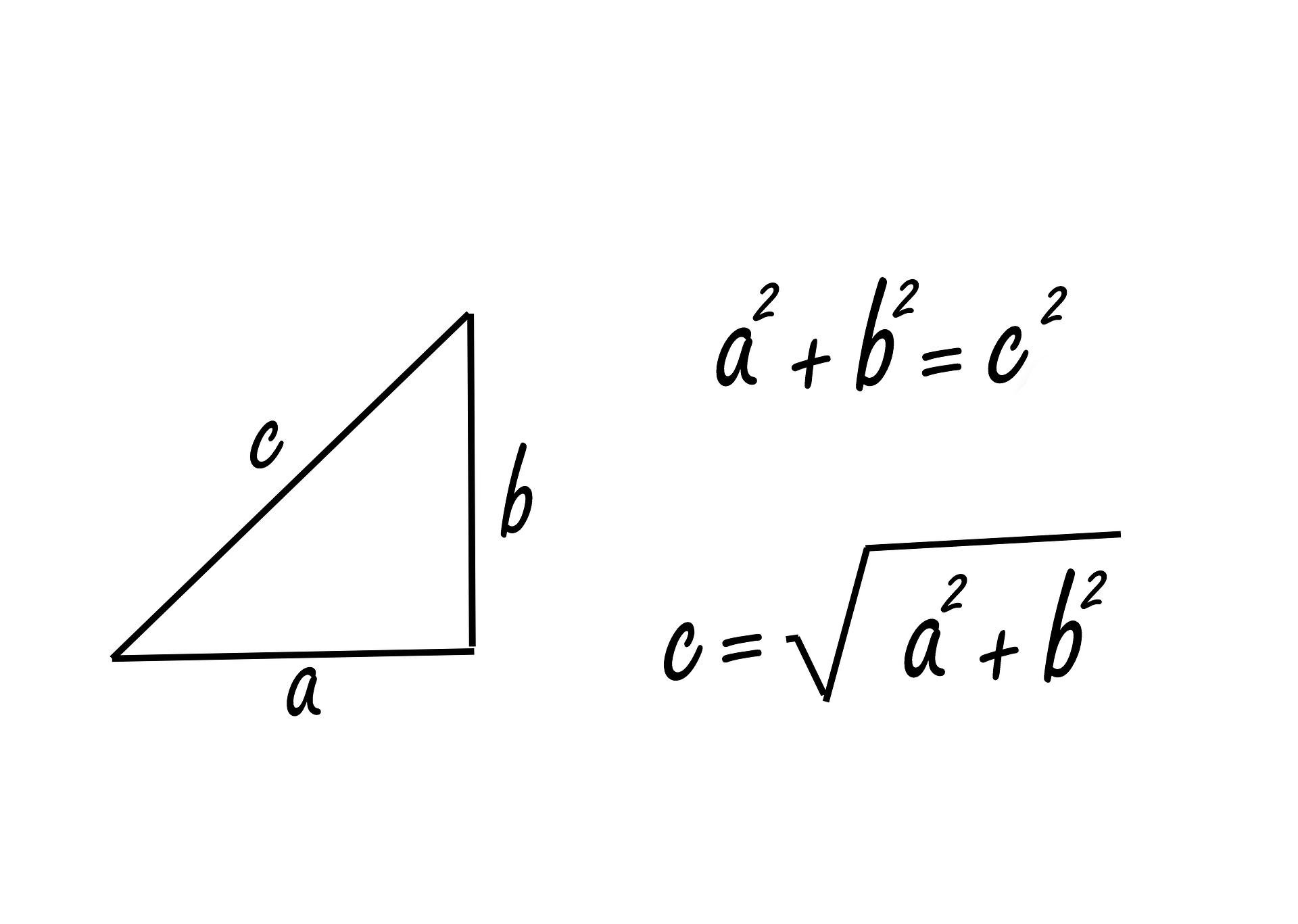# Trigonometry\$60.00
\$100.00

Dex Math provides a complete online Geometry course. Perfect for students who need to catch up on their Geometry skills, we offer a personal math teacher inside every lesson.

Algebra 2 is the third math course in high school and will guide you through among other things linear equations, inequalities, graphs, matrices, polynomials and radical expressions, quadratic equations, functions, exponential and logarithmic expressions, sequences and series, probability and trigonometry.

This Algebra 2 math course is divided into 13 chapters and each chapter is divided into several lessons. Under each lesson you will find theory, examples and video lessons.

What You'll Learn

• Numerical, graphical problems:
• Graphing trigonometric functions that involve amplitude, period, phase and vertical shifts,
• Algebraic properties od trigonometry:
• Develop algebraic understanding and skills that will help to solve equations concerning trigonometric functions,
• Right angle trigonometry:
• Understand the trigonometric functions that help in measurement and problem solving of the right-angled triangle.
• Law of sines
• Law of cosines
• Solving general triangles
• Inverse trigonometric functions
• Polar coordinates and Parametric equations
• Inverse trigonometric functions
• Trigonometric equations and identities
• Sinusoidal equations
• Sinusoidal models